Since they were first applied in spring-driven clocks in the 15th century to store mechanical energy, compression springs have evolved to have different shapes and designs. The conical spring is a type of compression spring that is now widely in a broad range of applications because of its unique design, which offers several advantages.

A conical spring is coiled helically in a decreasing (or increasing) outer diameter to form a conical or tapered shape. Unlike the regular compression springs with a constant diameter, a conical spring’s diameter is largest at the bottom and progressively reduces to the top.

This article will get back to the basics of conical springs. It will cover some of the advantages, applications, and design considerations for conical springs.

## What makes conical springs special?

When a conical spring is compressed, the coils collapse around themselves, with the smaller diameter coil (from the top) fitting into the next coil’s inner diameter. In addition, there is no contact of coils throughout the whole movement of the spring.

This unique attribute is called the nesting (or telescope) effect of conical spring, and it accounts for the several advantages that they offer. For example, conical springs are able to provide more rigidity and stability than normal compression springs when compressed. This is because their larger base diameter serves as a stable pod during operation.

Conical springs’ unique design and nesting ability help prevent buckling (bending of the spring) when they are compressed, making them ideal in applications where buckling is a serious concern. In addition, engineers can find conical springs useful in applications where there is a space limitation.

## Some key parameters for conical spring design

Consider a typical conical spring, as shown in the figure.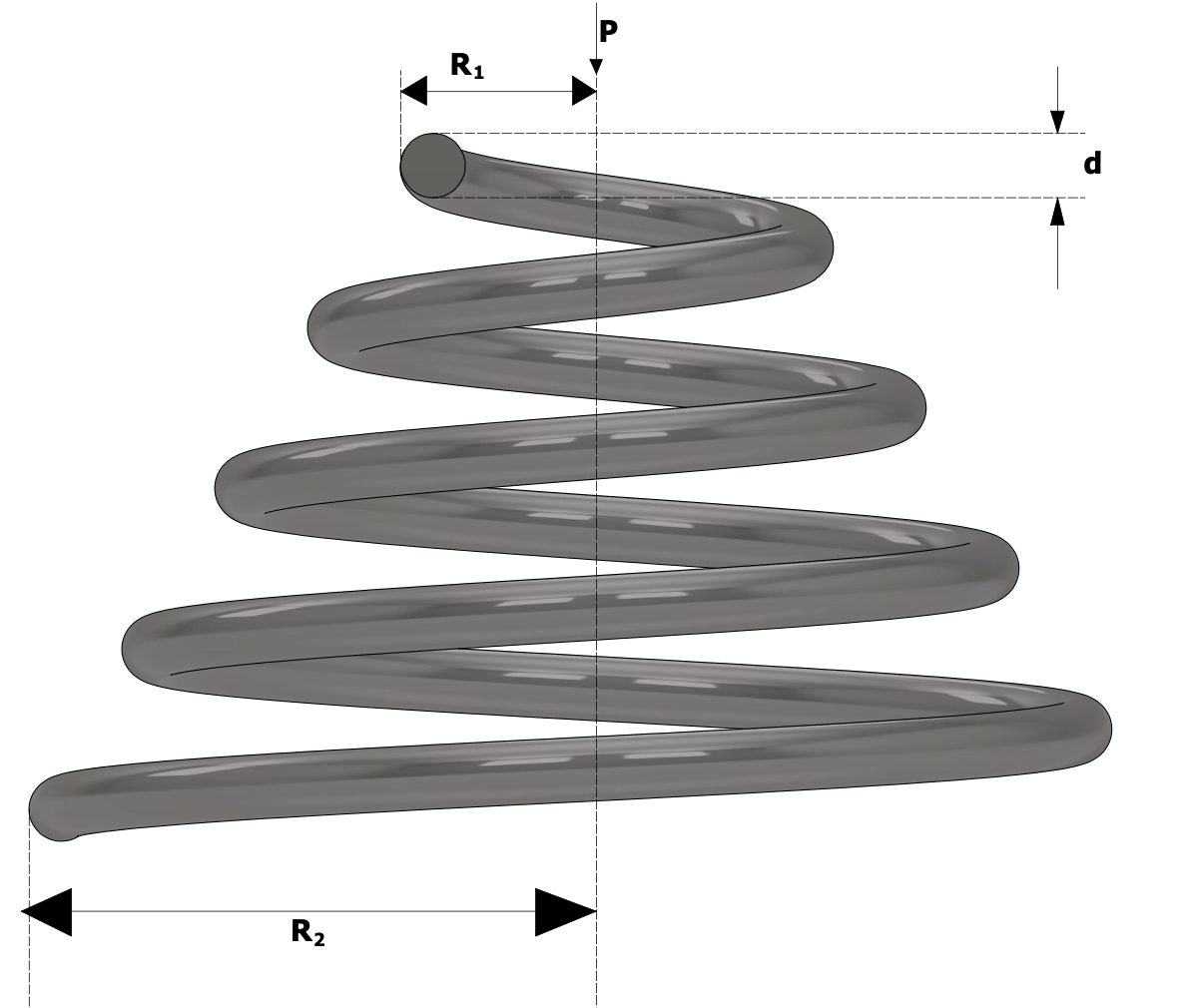Conical spring illustration.

When a load is applied to the spring, it compresses the spring, causing a slight deflection. This deflection can be estimated using: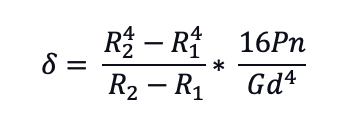Conical spring deflection equation.

Where:

R2 = Lower center radius of coil (mm)

R1 = Upper center radius of the coil (mm)

G = Shear modulus (N/m2)

d = wire diameter (mm)

The shear stress on the conical spring increases from the top to the bottom of the conical spring. The maximum stress occurs at the point of maximum coil radius (at the bottom of the conical spring). It can be obtained using: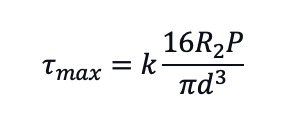Conical spring stress equation.

Consider the example of a conical spring made of high carbon steel with a shear modulus of 7.85 x 104 N/m2, a lower center radius of 10, and an upper center radius of 5. Suppose the total number of coils is 6, the total number of active coils is 4, the wire diameter is 1.5 mm, and the coil’s free height is 16 mm, the following design calculations will help calculate the allowable load at different spring heights.

When the spring is compressed to a spring height of 11 mm, the maximum allowable load on the spring can be calculated as follows: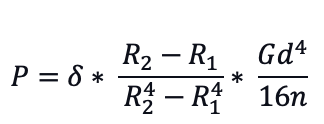Conical spring, maximum load equation 1.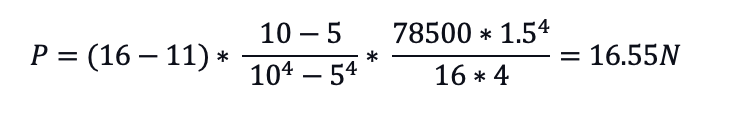Conical spring, maximum load equation 2.

## Conclusion

Conical springs offer any advantages if they are correctly sized for a particular application. While this article presents helpful information about conical spring designs, there are still several other things an engineer must consider when sizing conical springs. For example, there is still the need to determine optimum spring materials, solid length, among others. As such, engineers are advised to reach out to conical spring manufacturers to discuss their application requirements.